# Triangles - Perimeters and Areas

Go back to  'Mensuration'

The area of  a  triangle can be determined in a number of ways, based on what information  is available to us.

Consider the following  figure, which shows a triangle ABC with altitude AD equal to h units in length.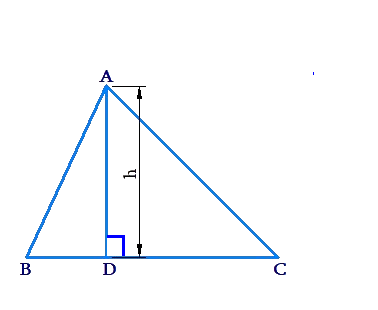The area of this triangle can be written as

\begin{align}&area\left( {\Delta ABC} \right) = \frac{1}{2} \times base \times height\\& \qquad\qquad\quad\quad\;= \frac{1}{2} \times BC \times h\end{align}

If we take a different side to be the base (say, AC),then we would  have to take the corresponding altitude (the altitude through B).

Suppose that the lengths of all the sides of a triangle are known, as shown in the following figure: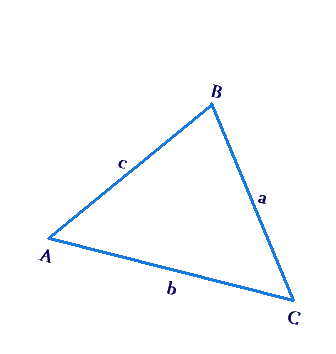Note the naming convention. The length of the side opposite to $$\angle A$$  is represented using the letter a, and so on.

The perimeter of this triangle is a + b + c. We define the semi-perimeter s as

$s = \frac{{a + b + c}}{2}$

In terms of s and abc, the area of the triangle can be expressed using Heron’s formula:

$area(\Delta ABC) = \sqrt {s(s - a)(s - b)(s - c)}$

Thus, in general, a  triangle’s area can be evaluated using one of the following two techniques:

•     $$\frac{1}{2} \times base \times height$$

•     $$Heron's\;{\rm{ formula}}$$

The  following are some specific scenarios involving  area calculation of triangles:

1. A right-angled triangle:

If two sides are known in a right -angled triangle, the third can be calculated using the Pythagoras Theorem. Consider  the following figure: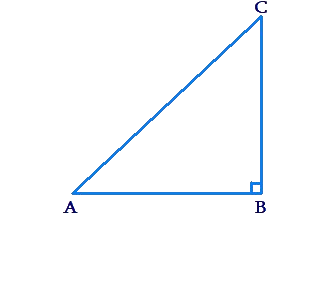We can write the areas of this triangle as

$area\left( {\Delta ABC} \right) = \frac{1}{2} \times AB \times BC$

2.  An Isosceles triangle:

In the following figure, $$\Delta ABC$$  is isosceles with AB = AC = a units and BC = b units: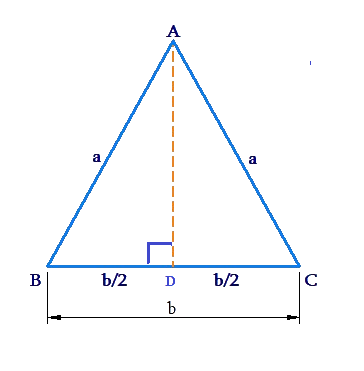We can write the area of this triangle as follows:

\begin{align}&area\left( {\Delta ABC} \right) = \frac{1}{2} \times BC \times AD\\ &\qquad\qquad\qquad\,\;= \frac{1}{2} \times b \times \sqrt {{a^2} - {{\left( {\frac{b}{2}} \right)}^2}} \\&\qquad\qquad\qquad\,\;= \frac{1}{4}b\sqrt {4{a^2} - {b^2}} \end{align}

3. An equilateral triangle:

The  following figure shows an equilateral triangle with each side equal to a units: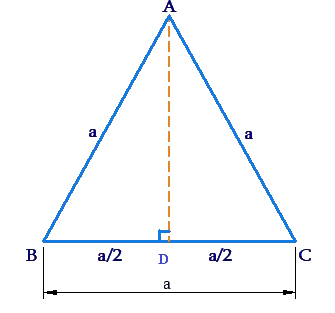We have

\begin{align}&AD = \sqrt {{a^2} - {{\left( {\frac{a}{2}} \right)}^2}} = \sqrt {\frac{{3{a^2}}}{4}} \\& \qquad= \frac{{\sqrt 3 a}}{2}\end{align}

Thus,

\begin{align}&area\left( {\Delta ABC} \right) = \frac{1}{2} \times BC \times AD\\& \qquad\qquad\qquad\;= \frac{1}{2} \times a \times \frac{{\sqrt 3 a}}{2}= \frac{{\sqrt 3 }}{4}{a^2}\end{align}

You  are urged to remember  this expression. For the area of an equilateral triangle.

Example 1: Find the area and perimeter of an equilateral triangle whose height is 10 cm.

Solution: Let the length of each of the triangle’s sides be a units. Consider the following figure: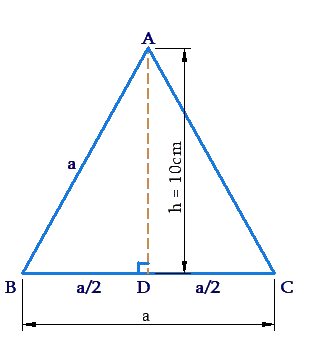We have in $$\Delta ABD$$:

\begin{align}&{a^2} = {h^2} + {\left( {\frac{a}{2}} \right)^2}\\& \Rightarrow \frac{{3{a^2}}}{4} = {h^2}\\& \Rightarrow a = \frac{{2h}}{{\sqrt 3 }}\end{align}

The area A  of the triangle is

\begin{align}&A = \frac{{\sqrt 3 }}{4}{a^2} = \frac{{\sqrt 3 }}{4} \times \frac{{4{h^2}}}{3}\\&\frac{{{h^2}}}{{\sqrt 3 }} = \frac{{100}}{{\sqrt 3 }}cm \approx ~57.7 \,\rm{cm^2}\end{align}

The perimeter of the triangle is

\begin{align}&P = 3a = 3 \times \frac{{2h}}{{\sqrt 3 }}\\&\quad = 2\sqrt 3 h = 20\sqrt 3 cm \approx 34.6\, \rm{cm}\end{align}

Example 2: The sides of a triangular park are in the ratio 12:17:25, and its perimeter is 1080 m. What is its area?

Solution: We assume the triangle’s sides to be 12 km, 17 km and 25 km for some constant k . We have:

\begin{align}&12k + 17k + 25k = 1080\\& \Rightarrow 54k = 1080 \Rightarrow k = 20\end{align}

The sides of the triangle are  thus:

240 m, 340 m, 500 m

Now, the semi-perimeter of the triangle is

$s = \frac{{1080}}{2} = 540m$

Using the Heron’s Formula, the area A of the triangle is

\begin{align}&A = \sqrt {540\left( {540 - 240} \right)\left( {540 - 340} \right)\left( {540 - 500} \right)} \\ &\quad= \sqrt {540 \times 300 \times 200 \times 40} \\& \quad= 36,000\, \rm{m^2}\end{align}

Example 3: Find the area of the shaded region in the  following figure: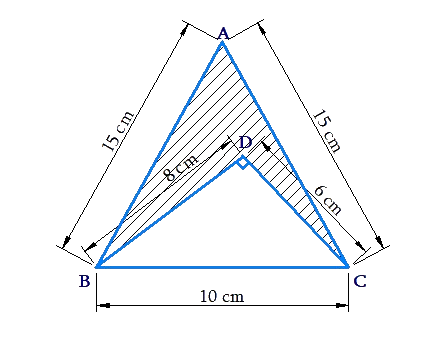Solution:  The altitude for $$\Delta ABC$$  is

\begin{align}&h \;= \sqrt {{{15}^2} - {5^2}} \left( {how?} \right)\\&\quad = 10\sqrt 2\, \rm{cm}\end{align}

Thus,

\begin{align}&area\left( {\Delta ABC} \right) = \frac{1}{2} \times BC \times h\\& \qquad\qquad\qquad\;= \frac{1}{2} \times 10 \times 10\sqrt 2 \\&\qquad\qquad\qquad\;= 50\sqrt 2 \,\rm{cm^2}\end{align}

Also,

\begin{align}&area\left( {\Delta BDC} \right) = \frac{1}{2} \times CD \times BD\\&\qquad\qquad\qquad\; = \frac{1}{2} \times 6 \times 8= 24\, \rm{cm^2}\end{align}

Thus, the area A of the shaded region is

\begin{align}& A \;= \rm{area}(\Delta ABC) - area(\Delta BDC)\\&\quad = (50\sqrt 2 - 24)\,\rm{cm^2}\\&\quad \approx 46.7\, \rm{cm^2}\end{align}

Mensuration
grade 9 | Questions Set 1
Mensuration
Mensuration
grade 9 | Questions Set 2
Mensuration